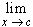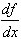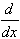#Interactive Real Analysis

Next | Previous | Glossary | Map

## 6.5. Differentiable Functions

### Definition 6.5.1: Derivative

Let f be a function with domain D in R, and D is an open set in R. Then the derivative of f at the point c is defined as
f'(c) =If that limit exits, the function is called differentiable at c. If f is differentiable at every point in D then f is called differentiable in D.

Other notations for the derivative of f areorf(x)

Next | Previous | Glossary | Map